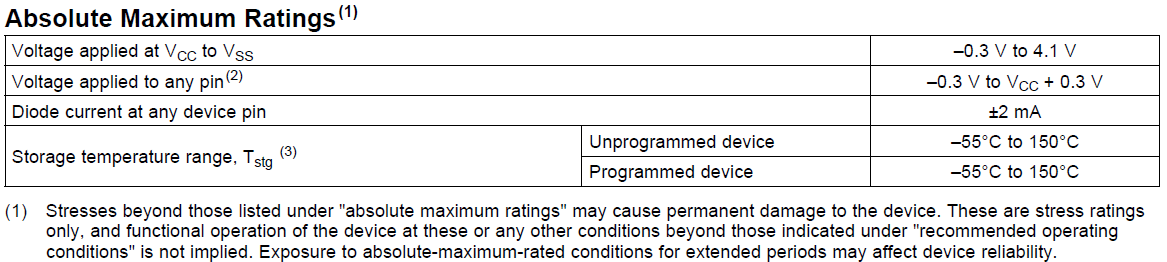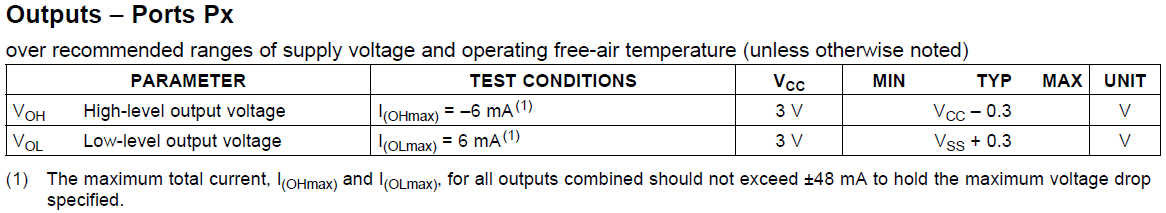# Microcontroller GPIO Protection Tutorial

Microcontroller GPIO protection is an essential element of any circuit design.

GPIO ports generally have maximum voltage and current ratings, if these are exceeded it can result in the microcontroller being damaged or completely destroyed.

Therefore it is important to use external circuitry to protect the ports from excessive voltage, or excessive current being sourced from a pin.

The principles used in this post are for the MSP430G2231, however they can be applied to Arduino, Raspberry Pi and other microcontrollers, by taking into account the voltage and current specifications for each microcontroller, then changing the zener diode and resistor value accordingly.

## Over Voltage Protection

This is fairly easy to achieve with some basic external components, to explain this a simple example will be used based on the Texas Instruments MSP430G2231.  The maximum ratings for the integrated circuit need to be determined, this information can be obtained from the devices datasheet, MSP430G2231 datasheet can be found here.

The image below shows an extract from the MSP430G2231 datasheet, the maximum voltage rating can clearly be seen as 4.1V, there is also a cautionary warning which should be noted.Now lets assume we are using one of the ports as an ADC input for a sensor, the voltage range for the ADC input on the MSP430G range is 0V to 3V.  So now we know the operating range and the maximum voltage, we can design some simple interface circuitry to ensure the microcontroller is protected.

The schematic diagram below shows a basic circuit which could be used to protect against over voltage.

The main components here that provide the over voltage protection, are D1 and R3.  D1 is a 3V3 Zener diode, which clamps the voltage if it exceeds 3.3V.  R3 has a dual purpose firstly if the voltage exceeds 3.3V the excess voltage will be dropped across the resistor, as the diode will have 3.3V across it i.e. over voltage is 5V, R3 will have 1.7V across it and D1 will have the remaining 3.3V across it.  R3 also acts as a current limit resistor, and Ohm’s Law can be used to calculate the maximum current with a known voltage.  Something to note here is the zener diode will take approximately 5mA of current in normal operation, this is less than ideal for battery operated applications.The other components R1, R2 and the opamp are here to simulate a dummy sensor input.  However by designing a circuit using a single rail to rail opamp, the output of the opamp cannot exceed it’s rail to rail voltage, so with a supply of 0 to 3.3V the output cannot exceed 3.3V.  Therefore using a rail to rail opamp in a configuration similar to the one shown, can eliminate the need for the zener diode altogether.

## Over Current Protection

Over current protection as with over voltage protection, is quite simple to achieve.  Again it is best to consult the datasheet for the device to determine the current ratings, as can be seen below in an extract from the MSP430G2231 datasheet.So the maximum recommended current that can be sourced or sunk is 6mA, and the total maximum current for all ports is 48mA (MSP430G2231 has 10 GPIO pins in total).  There are some further graphs shown in the datasheet, these show that it is possible to source 8mA from a GPIO pin, but the voltage on this pin will drop to approximately 2.7V as a result of the high current.  I would not recommend placing a load which draws 8mA, especially not on more than 1 pin, I always try to keep the current drawn from a GPIO around 1mA in most circumstances.  We can also see the voltage from the GPIO pins will be 3V.

By placing a resistor directly connected to the GPIO pin before any additional circuitry, we can limit the amount of current being sourced from the pin.  This resistor can be calculated by taking the 3V provided by the GPIO pin, lets assume we want to limit the current to 1mA, this gives us a value of 3kΩ the nearest standard value (going up) is therefore 3.3kΩ. This calculation can also be reversed and used to prevent excessive currents being sunk by the microcontroller.

3V rated at 1mA is not going to give much scope for driving external circuitry i.e. an LED or a relay, so it is best to use this to drive a transistor, using the transistor as a switch.

I take great care when writing all the tutorials and articles, ensuring all the code is fully tested to avoid issues for my readers.  All this takes time and a great deal of work, so please support the site by using the Adfly links etc.  If you have found this useful or have any problems implementing, please feel free to leave a comment and I will do my best to help.